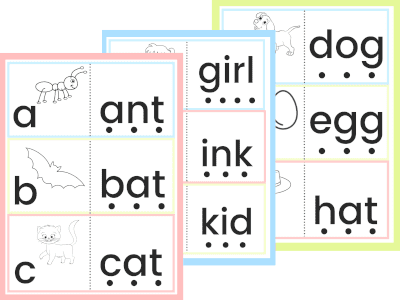# Addition Activity with Climbing Squirrels

5
670 views

This climbing squirrel addition activity makes doing those first sums so much fun, kids won’t even notice they’re using a number line.

Number lines are a commonly used in schools to help children understand how to add 2 numbers together.

The line allows them to first count up to one number. They can then start from that number on the line and count on from there for the second number. The final number that they reach on the number line is the sum of those two numbers.

By giving children a visual way of seeing these sums, children can understand the process of adding two numbers together. And see how many acorns the squirrel can gather up too!

### Summary:

Time: 10 minutes
Ages: Big kids
Difficulty to make: Easy peasy
Skill: Addition activity using a number line

1

1

## You will need:

If you prefer to print each page individually, you can click on each page link below:

22

### Step 1: Print out the activity mats and cut out the squirrel and acorns

Print out the 4-page activity pack for this game. Click on the link below to view and print:

Cut out each of the 12 acorns shown here. These are what the squirrel will be gathering.

On the page with the large bag, there is a squirrel image above it. This will be your marker so cut it out as well.

33

### Step 2: Throw the dice and move the squirrel

Place your squirrel at the bottom of the tree trunk and throw your two dice.

Ask your child to look at the first dice. How many dots are there?

Move the squirrel up the tree trunk to that number. Here the squirrel has climbed up to number 4.

44

### Step 3: Continue counting on from that number

Ask your child to look at the second dice. How many dots are there? What number is that?

Starting from its position after the first number, they can now move the squirrel further up the tree trunk by the number of spaces shown on the second dice.

In this case a number 5 was thrown. The squirrel was on number 4 and has moved up 5 spaces. It has now reached the number 9.

Point out that they have worked out the sum. First they moved the squirrel to up to number 4. Then they moved it 5 spaces more and reached the number 9. So 4 and 5 is 9.

You can say the addition sum out loud: 4 and 5 is 9.

55

### Step 4: Fill up their bag with acorns

For each sum you can also count out the acorns. First count out the number shown on the first dice. Place them in the squirrel’s bag.

Then count out the number shown on the second dice. Place them in the bag too.

How many acorns has he collected in total? Is this the same as the number the squirrel has reached on the tree trunk?

Repeat the game several times moving the squirrel up the number line and saying the addition sum together.

You can count out the acorns each time as well to reinforce the sum.

66

### Step 5: Write out the sums

After your child is familiar with the concept of combining two numbers together, you can introduce the word ‘plus’.

‘Plus’ may be a new word for them, so it’s best to introduce it when they understand the concept of adding numbers together using everyday language (for example 4 and 5 together make 9).

Explain that ‘5 and 4’ can also be said as ‘5 plus 4′. The symbol for ‘plus’ is ‘+’. The symbol for ‘is’ would be ‘=’.

Show your child how to write out 5 and 4 as a maths sum. It would be 5 + 4 = 9.

Each time your child moves the squirrel up their number line, you can now ask them to write out the sum on the worksheet.

They start by writing the numbers shown on each dice. Then they move their squirrel on up by both numbers, until they get the total which they can then write in the box.

If you play this game again and again your child will soon realise that they don’t have to count out the first number in the addition sum.

They can simply start at this number and count on to find the answer. Using a number line helps them visualise what they are doing each time they tackle a simple addition sum.

Practising with number lines helps to give them those first building blocks in maths. They learn to understand what it means to add two numbers together, and to learn common sums off by heart.

This will help them to visualise sums in their minds in the future, with mental maths.## Get FREE Phonics Flashcards

Grab our phonics letter pack today!